99's substitutes

Manufacturing electronic tubes replacements14. Testing the first ersatz: LO verification

As I have made only one substitute, I decided to test it in the local oscillator stage.
The operation is easy: plug in the power supplies, and a oscilloscope on the oscillator coils.
However, it should be noted an important point: as the test is done with only one lamp, you either limit the heating voltage ( "A"), either limit the current with a 22 ohm resistor in the circuit.
Without this precaution, there is a risk of burning the filament of the lamp!
Here's how to connect the power supplies (no need to connect the +45 V):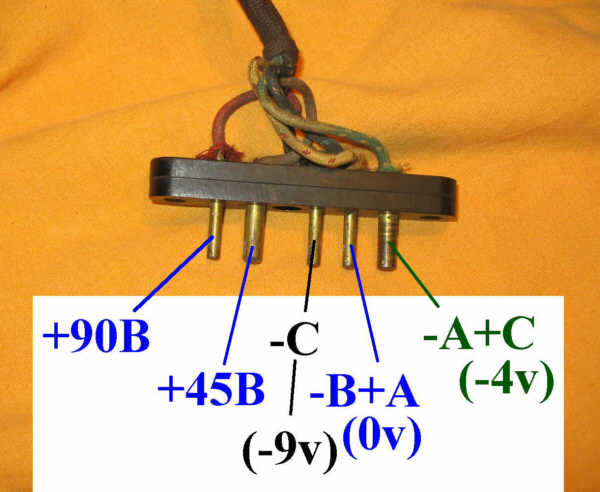The oscilloscope can be connected to pin #5 of Catacomb:The lamp is plugged in slot #2: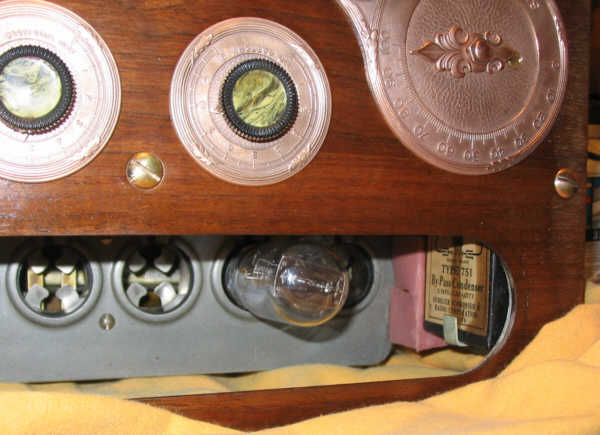Here waveform when the varcap is maxi: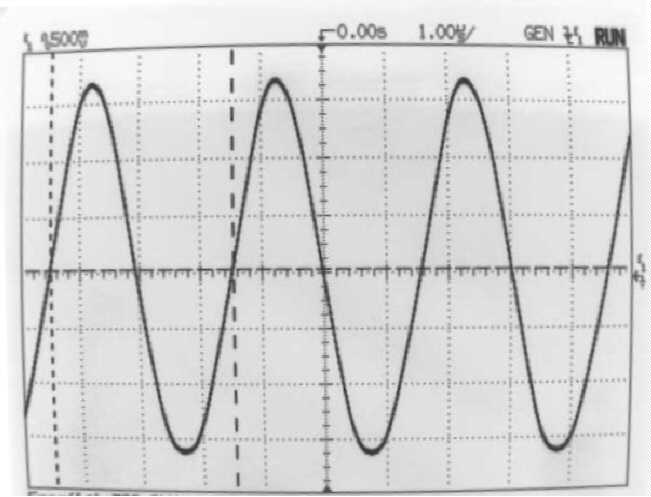The frequency is 239kHz .... very strange ...

On the other hand, when the varcap is mini, the oscillation does not occur; If we want the OL works well, varcap tuning must be at least 10%, in this case, the maximum frequency is 543kHz.
Is there any problem?

15. Wiring another ersatz

During ersatz tests on RCA radiola III, I tried a 1L4 with success...
Maybe this lamp works too?
Wire it in triode mode (G2 = A) and adjust the heating voltage (1.4 V)
I decided to quickly wire an adapter, which is to say that I use a 7-pins miniature socket wired with a 4-nubs base, in this way, the lamp becomes replaceable.
Here the lamp, ready to be tested:16. Testing this second lamp on the LO stage

The results are identical: same frequencies. Just a small difference: this lamp does not allow -10V for -C bias, it requires minimum -7V. Otherwise, the oscillation does not start ever.

So, this proves that whatever the lamp, the oscillation occurs at really low frequencies .
Pondering a little, could we not imagine that the use of second harmonic of the LO produces the beat?

Consider the numbers:
varcap mini: Fmax = 587kHz (with the 1L4) multiplied by 2 = 1174 kHz
varcap maxi: Fmin = 239kHz multiplied by 2 = 478kHz

Assuming that we take the lower beating, it means that the wavelengths that can be received will be fixed between:
300000 / (1174 +42) equals 247 and 300000 / (478 +42) equals 577 meters.
Values that I think are quite acceptable.
To make sure it is the #2 harmonic which is used, I can measure the inductance of the coils of the local oscillator ...

To do this, simply unplug the 3 wires of the coils from the Catacomb, and the wire on the varcap too.
Then input a signal on the non-tuned coils, and visualize the signal on the other coils, tuned with fixed known value capacitor, then tuned with 2 identical caps (or one with double value).
Thus, through this little spreadsheet file, with 2 measures, I can determine inductance and capacitance of the coil.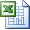The coil inductance

The inductance is approximately 550 µH. The capacitance is around 30pF, that gives, with varcap tunde on max, a frequency about 250kHz, which is consistent with what I found.

Thus, by design, it is truly the second harmonic beating with the incident wave to produce the 42kHz IF.

17. Constructing other substitutes

I tried several other lamps tu substitute 99.

First, a 3S4 that has not yielded good results in LO stage,
then a russian 2SH27L which gives good results.

I made a 2° ersatz of the first type (with a 3Q5), and then, surprise: no more oscillation! I had to remove the 180k resistor, i.e. wire it in truly triode mode.

Then I get a 5° version that works well.

Summarize:

• "99-A" based on 3Q5, worse than version -E
• "99-B" based on 1L4
• "99-D" based on 2SH27L
• "99-E" based on 3Q5, best version.
• ("99-C" is abandoned)

So I built a total of 6 ersatz:

• one -A
• two -B
• two -D
• one -E

Here is a picture of the lamps set: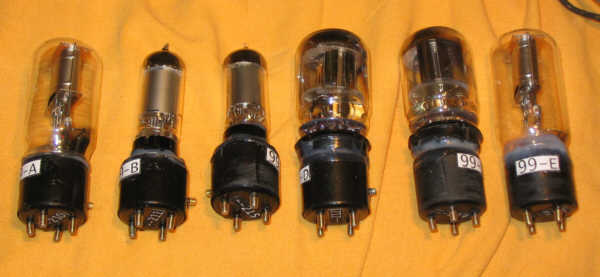And the outlines of the 3 versions that I keep now: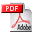99's substitutes